# Past Paper Maths 5th Class 2008 Punjab Board (PEC) Solved Paper

1
530

Past Paper Maths 5th Class 2008 Punjab Board (PEC) Solved Paper

It is a 5th class Maths paper of 30 multiple choice questions and 4 open ended questions. It is an Urdu Medium variation of Maths paper.  Multiple questions are very tricky. Some of them are as follows:

• What is the next figure of 1,99,999?
• What is largest figure from 0,1,4,5,6,7
• What is the result if we add .009 in 1.991?
• The price of 50kg sugar is 1500. What will be the price of 1kg?
• Kashif pays Rs.2.5 zaqat on Rs.100. What will he pay zaqat on Rs.400?
• What is answer of 3+4 x5?
• If price of 10 copies is Rs.105, then what will be the price of one copy?
• The monthly rent of a house is Rs. 1,200. What will be annual rent of that house?
• What are parallel lines?
• What is rectangle?
• Divide 2.4 with 0.8

First part of 31s question i.e. first open ended question is easiest question. It is a simple multiply question.  The second part of question 31 is a statement question.  Question number 32 is from algebra and question number  33 is a conversion question. Have a look at the solution of all these questions.

Objective Type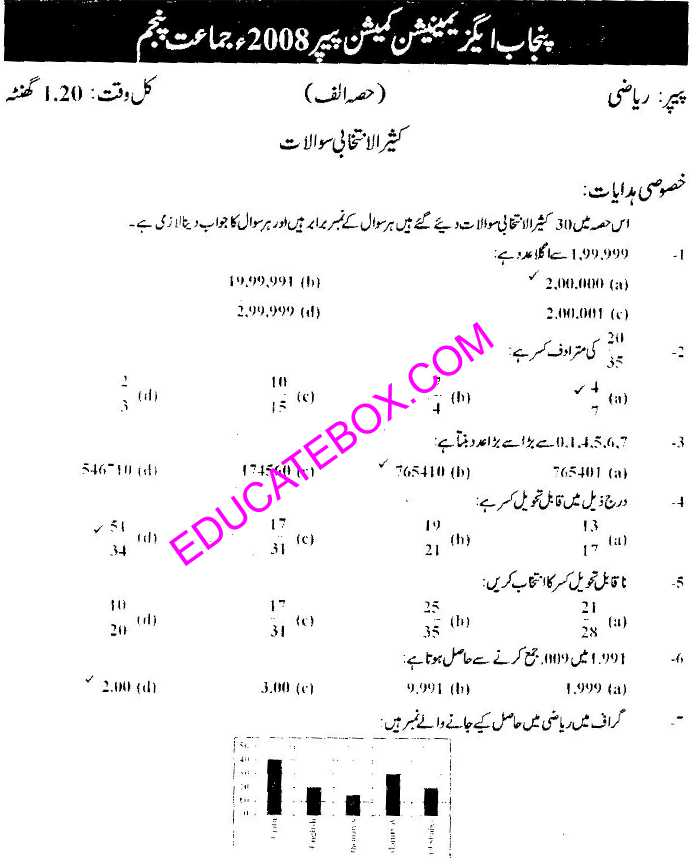Past Paper Maths 5th Class 2008 Punjab Board (PEC) Solved Paper -Page2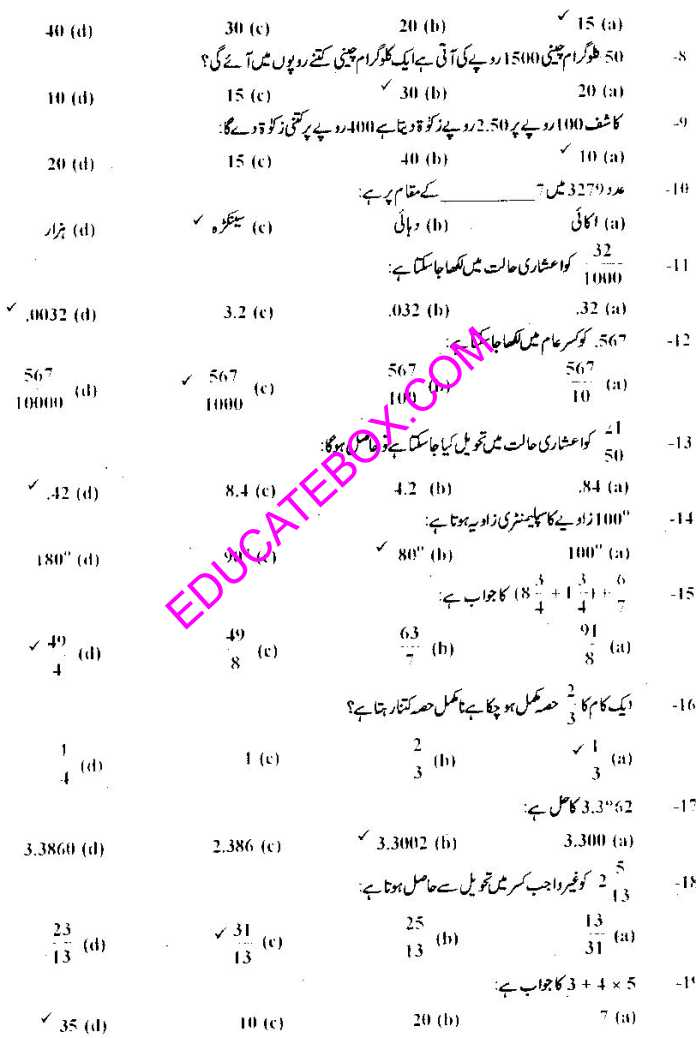Past Paper Maths 5th Class 2008 Punjab Board (PEC) Solved Paper -Page 3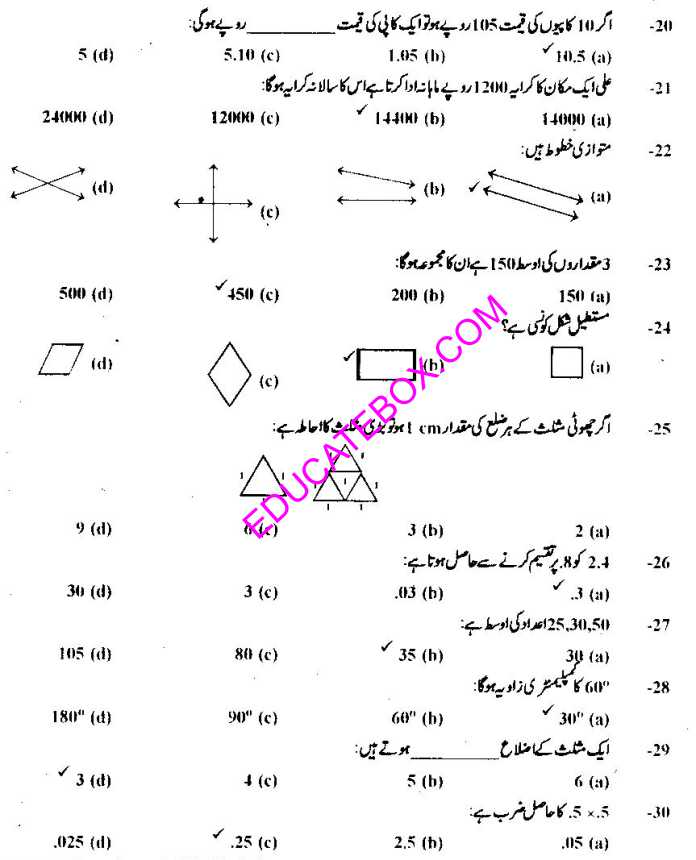Past Paper Maths 5th Class 2008 Punjab Board (PEC) Solved Paper -Page 4

Subjective Type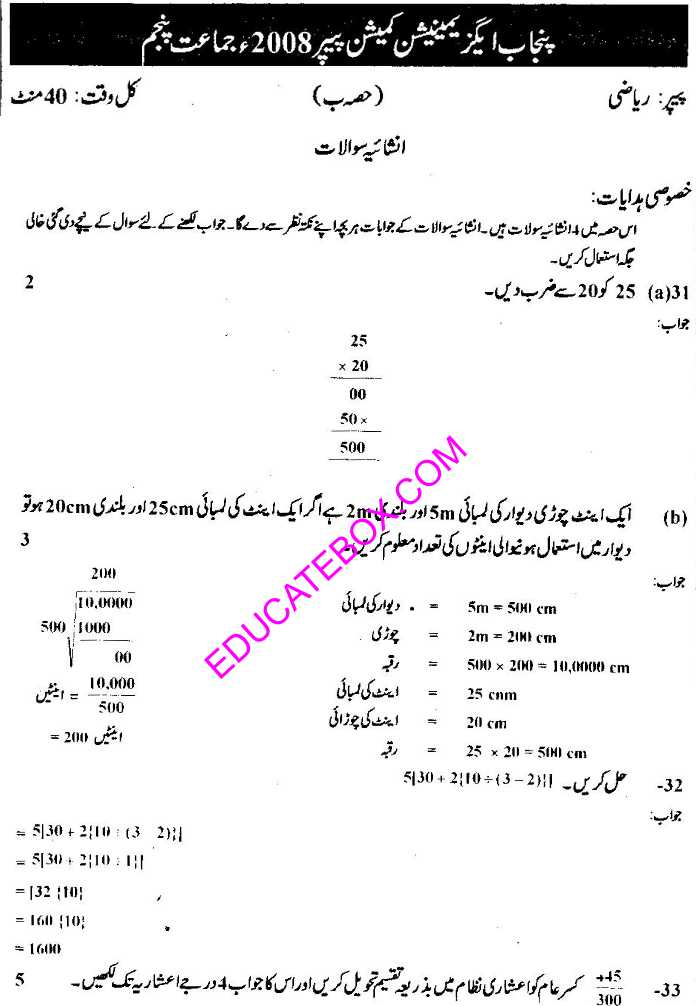Past Paper Maths 5th Class 2008 Punjab Board (PEC) Solved Paper -Page 5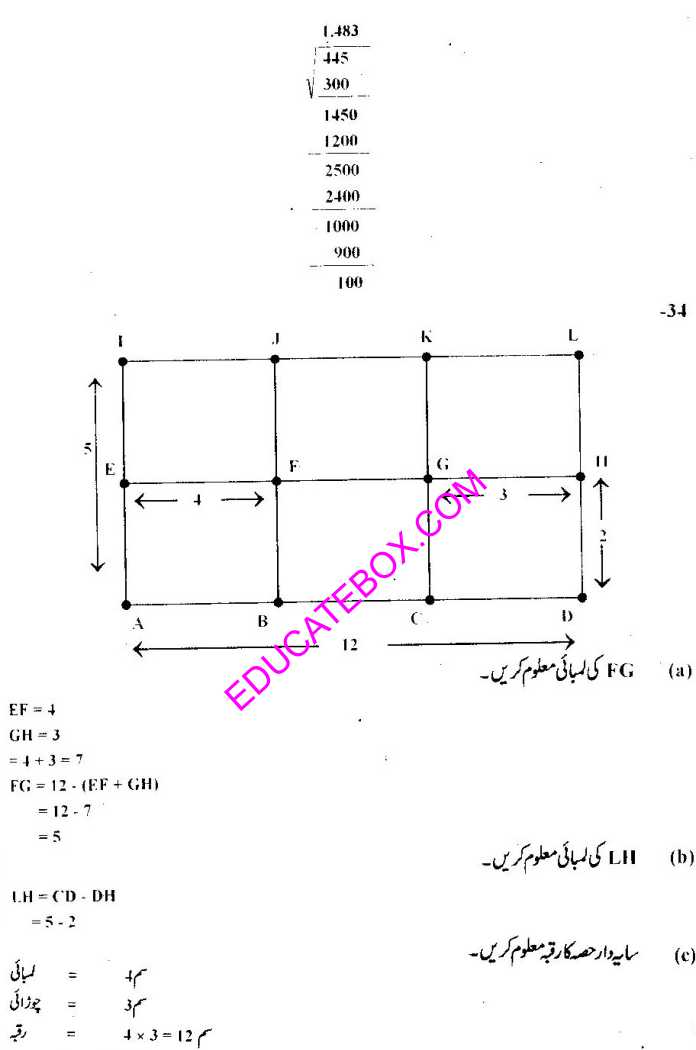#### 1 COMMENT

1.Nagesh

Past papers of previous years with relevant source materials would help the students to achieve excellent marks.